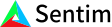1. Bob Anderson
×
IntuitiveML

## Intuition: What is Accuracy, Precision, and Recall in machine learning, and how do they work?

Jun 18, 2020

You have a model, and now you want to judge how well it performs. How do you measure model effectiveness?

There are several metrics you could use to judge how good a classification model is, the most common of which are accuracy, precision, and recall. Accuracy measures how much of the data you labeled correctly. That is, accuracy is the ratio the number labeled correctly over the total number. If you are trying to classify just one thing (e.g. hot dog or not), accuracy can be written as (the number of true positives + the number of true negatives)/(number of true positives + number of true negatives + number of false positives + number of false negatives). True positives are examples with a positive label that you labeled as positive, e.g. you labeled a hot dog as a hot dog. Similarly, true negatives are examples with a negative label that you labeled as negative, e.g. you labeled a cat as not a hot dog. On the other hand, false positives are examples that were negative that you labeled positive, e.g. you labeled a cat as a hot dog (how could you!?) and similarly false negatives are examples that were positive that you labeled negative, e.g. you labeled a hot dog as not a hot dog. The true or false in true positive, false negative, etc., indicates whether you labeled it correctly or not, and positive or negative is what you labeled it. So accuracy is just the number of things you correctly labeled as positive and negative divided by the total number of things you labeled. In the case where you are trying to classify a lot of things instead of just one, the overall accuracy is just the number of things you correctly labeled in each category divided by the total number of things you labeled.

Precision is a measure that tells you how often that something you label as positive is actually positive. More formally using the notation from earlier, precision is the number of true positives/ (the number of true positive plus the number of false positives). On the other hand, recall is the measure that tells you the percentage of positives you label correctly. That is, recall is the number of true positives/(the number of true positives plus the number of false negatives). The difference between precision and recall is kind of subtle, so let me reiterate: precision is the number of positive examples you labeled correctly over the total number of times you labeled something positive, whereas recall is the number of positive examples you labeled correctly over the total number of things that were actually positive. You can think of precision as the proportion of times that when you predict its positive it actually turns out to be positive. Where as recall can be thought of as accuracy over just the positives – it’s the proportion of times you labeled positive correctly over the amount of times it was actually positive.

In the multi-label case, precision and recall are usually applied on a per category basis. That is, if you are trying to guess whether a picture has a cat or dog or other animals, you would get precision and recall for your cats and dogs separately. Then it’s just the binary case again – if you want the precision for cats, you take the number of times you guessed correctly that it was cat / the total number of times that you guessed anything was a cat. Similarly, if you want to get recall for cats, you take the number of times you guessed correctly it was a cat over the total number of times it was actually a cat.